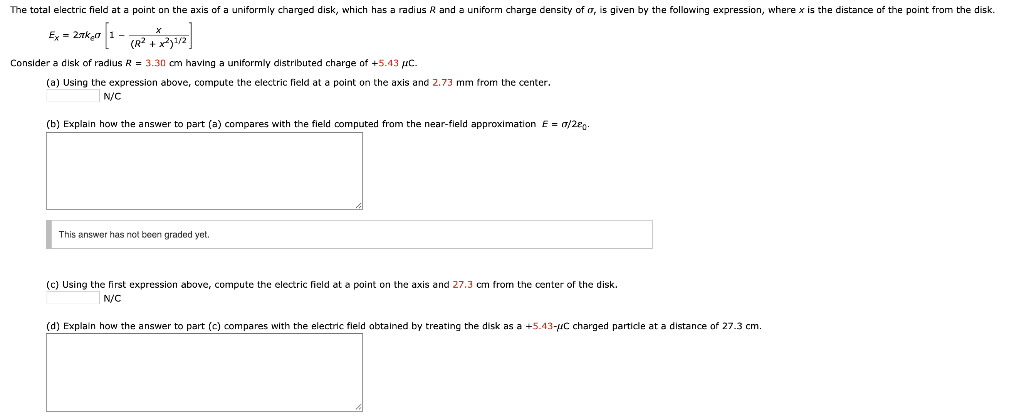Home / Answered Questions / Other / the-total-electric-field-at-a-point-on-the-axis-of-a-uniformly-charged-disk-which-has-a-radius-r-and-aw889

# (Solved): The Total Electric Field At A Point On The Axis Of A Uniformly Charged Disk, Which Has A Radius R An...The total electric field at a point on the axis of a uniformly charged disk, which has a radius R and a uniform charge density of O, is given by the following expression, where x is the distance of the point from the disk. Ex = 2720 1 - 2 Consider a disk of radius R = 3.30 cm having a uniformly distributed charge of +5.43 C. (a) Using the expression above, compute the electric field at a point on the axis and 2.73 mm from the center. N/C (b) Explain how the answer to part (a) compares with the field computed from the near-field approximation E = 7/2â‚¬. This answer has not been graded yet. (c) Using the first expression above, compute the electric field at a point on the axis and 27.3 cm from the center of the disk. N/C (d) Explain how the answer to part (c) compares with the electric field obtained by treating the disk as a +5.43-4C charged partide at a distance of 27.3 cm.

We have an Answer from Expert